# Team:UESTC-software/Model

threeModeling

Our project is mainly centered on how to improve the storage density and avoid the mistakes that may occur during the process of designing a stable, high-density DNA information storage system. There are two main technological processes, encoding and decoding, in the system.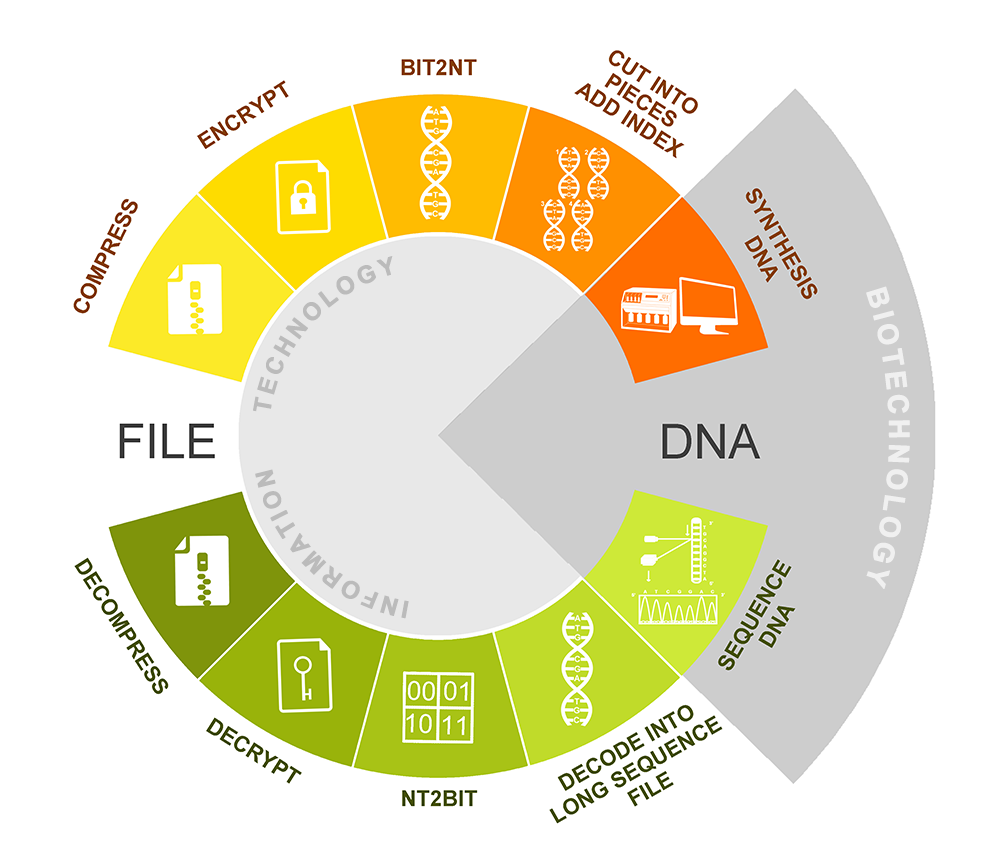Fig.1.system flow diagram.

## Encoding

Compression: bzip2 algorithm 

We used bzip2 algorithm which renowned as a high-quality data compression algorithm to compress the file. It typically compresses files to within 10% to 15% of the best available techniques, whilst being around twice faster at compression and six times faster at decompression. After this process, we get a bz2 compression file.

Encryption: ISAAC64 encryption algorithm 

Next, we use ISAAC64 encryption algorithm to encrypt the bz2 file. After you input your own password, ISAAC generates a pseudorandom stream of bits (a keystream). As with any stream cipher, these can be used for encryption by combining it with the plaintext using bit-wise exclusive-or; decryption is performed the same way (since exclusive-or with given data is an involution). After this process, we can get a sufficiently random binary file.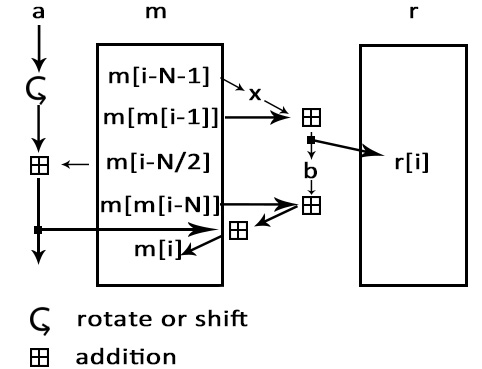Fig.2. encryption process.

Bit-to-nt conversion: quanternary system

In bit-to-nt conversion process, we use the idea of quaternary system. As we all know, there are four basic groups—A, T, C, G, and it can be seen as a quaternary system. In bit-to-nt conversion, one byte of bits converts into four bytes of A, T, C, G, using the scheme illustrated in Table 1.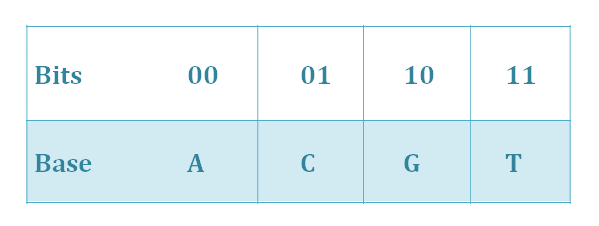Tab.1.Bit-to-nt conversion.

We read out the binary string S0 of the binary file generated in the last process. Use bit-to-nt conversion to convert S0 into a DNA string S1. We get a long DNA sequence.

Fragmenting & indexing

Write len( ) for the function that computes the length of a string, and define n=len(S1). Represent n in base-4 and prepend ‘0’s to generate a string S2 of quaternary such that len(S2)=15. Form the string concatenation

S4=S1.S3.S2(1)

(the symbol ‘ . ’ means the connection of two strings)

where S3 is a string of at most 49 ‘0’s chosen so that len(S4) is an integer multiple of 50.

Convert S2 and S3 to DNA strings S2’ and S3’using the scheme illustrated in Table 2.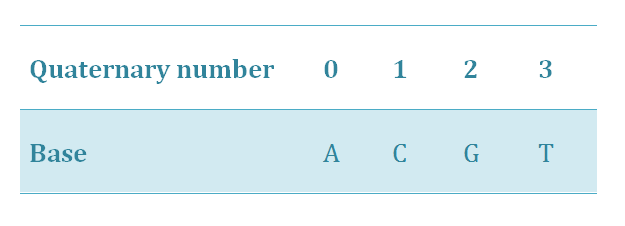Tab.2.Quaternary-to-nt conversion.

Recode the DNA string S3’.S2’ from the second character to S2’’ with repeated nucleotides as few as possible using the scheme illustrated in Table 3.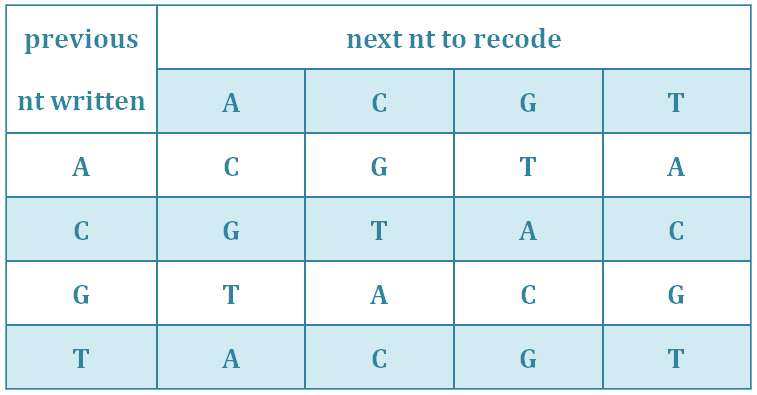Tab.3.Recoding table.

From

S5=S1.S2’’(2)

Define N=len(S5). Split S5 into overlapping segments of length 200 nt, each offset from the previous by 50 nt. This means there will be N/50-3 segments, conveniently indexed

i = 0 , 1······, N/50-4 (3)

segment i is denoted F_i and contains (DNA) characters

50i, …, 50i+199(4)

of S5. If i is odd, reverse complement Fi.

Let i4 be the base-4 representation of i, appending enough leading ‘0’s so that

len(i4)=12(5)

Recode i4 using the same strategy in Table 2 & Table 3 above, and i4 is represented in nt.

Even-odd check

Compute P as the sum(mod 4) of the even-positioned quaternary in i4.

P=(i42+i44+i46+i48+i410+i412)mod 4(6)

P acts as a ‘parity quaternary’—analogous to a parity bit—to check for errors in the encoded information about i.Form the indexing information string

IX=i4.P (7)

segment i is denoted Fi and contains (DNA) characters

50i, ......, 50i+199(8)

of S5. If i is odd, reverse complement Fi.

Let i4 be the base-4 representation of i, appending enough leading ‘0’s so that

len(i4) = 12(9)

Recode i4 using the same strategy in Table 2 & Table 3 above, and i4 is represented in nt.

Compute P as the sum(mod 4) of the even-positioned quaternary in i4.

P = (i42+i44+i46+i48+i410+i412 ) mod 4(10)

P acts as a ‘parity quaternary’—analogous to a parity bit—to check for errors in the encoded information about i.Form the indexing information string

IX = i4.P(11)

(comprising 12+1=13 nt)

Append the DNA-encoded version of IX to Fi to give indexed segment Fi’.

Direction tag

Then form Fi’’ by prepending A or T and appending C or G to Fi’ —choosing between A and T, and between C and G, randomly if possible but always such that there is no repeated nt. This ensures that we can distinguish a DNA segment that has been reverse complemented during DNA sequencing from one that has not—the former will start with G|C and end with T|A; the latter will start A|T and end C|G.

The segment Fi’’ are synthesized as actual DNA oligonucleotides and stored, and may be supplied for sequencing.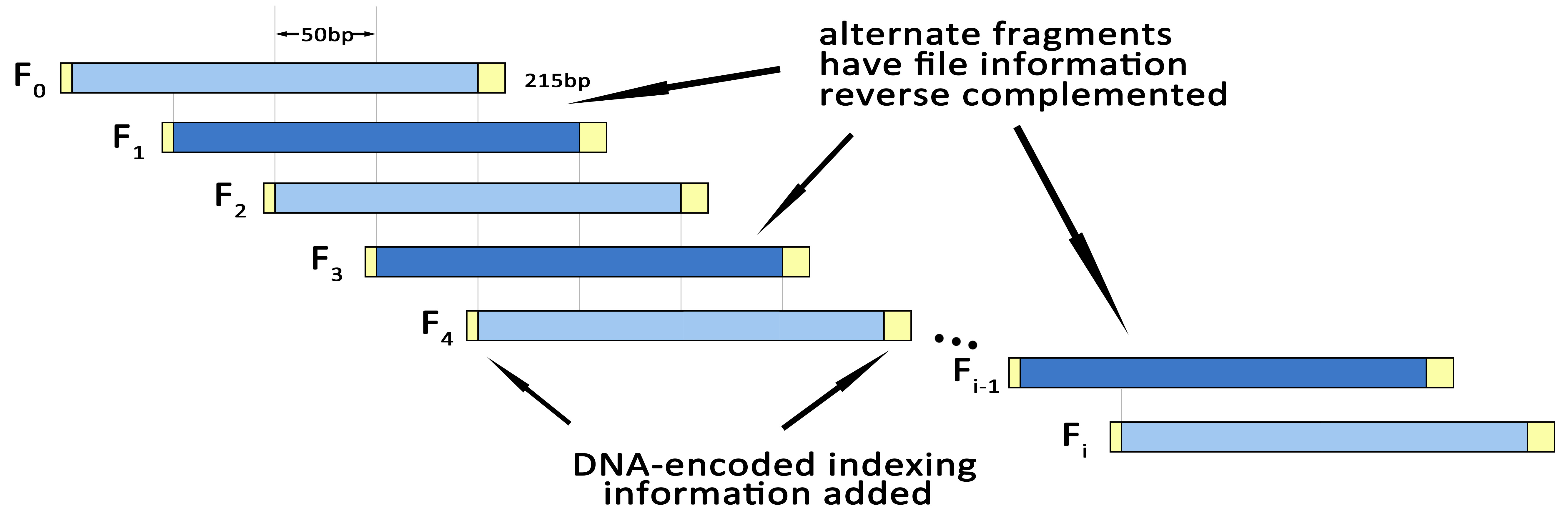Fig.3.Fragmenting & Indexing.

Safety testing: BLAST

In order to check whether the sequences we generated are safe, we use BLAST for sequence alignment. We do sequence alignment via the NCBI database to ensure accuracy. At the same time of generating the DNA sequences file, we also provide the BLAST report for downloading.

## Decoding

Recognition of the front and the end of a sequence

Reverse complementation during the DNA sequencing procedure(e.g. during PCR reactions) can be identified for subsequent reversal by observing whether fragments start with A|T and end with C|G, or start with G|C and end with T|A.

With these two ‘orientation’ nt removed, the remaining 213 nt of each segment can be split into the first 200 ‘message’ nt and the remaining 13 ‘indexing’ nt. Decode the ‘indexing’ nt to quanternary using the reverse of the encoding tables in in Table 2 & Table 3 above, and use P to check the correctness of i4.

Correction of segments through four-fold redundancy

Use i to determine the location of each fragment. Split each fragment into segments of length 50 nt. As we provide a four-fold redundancy, we compare the 50-nt segments which are in the same location and inaccurate bases can be corrected by using majority vote. Connect all the segments to a whole DNA sequence.

Decoding, decryption and decompression

Decode the DNA sequence by using the reverse of the encoding table in Table 1 above, and use ISAAC64 to decrypt it, and then decompress. We get the original file.

Fuzzy matching with high-throughput sequencing

Errors introduced during DNA synthesis, storage or sequencing could lead to various nt insertion, deletion or substitution. Recovery of information from fragments with such errors may be possible via PCR amplification and high-throughput sequencing.

## Example

In order to make our encoding process more understandable and clearer, we show an example here.

With a given text file, we read out its binary file, then compress it with bzip2 algorithm, and use a pseudorandom stream of bits to encrypt it. Then use bit-to-nt conversion to convert the binary numbers to bases. Shown in Figure 4.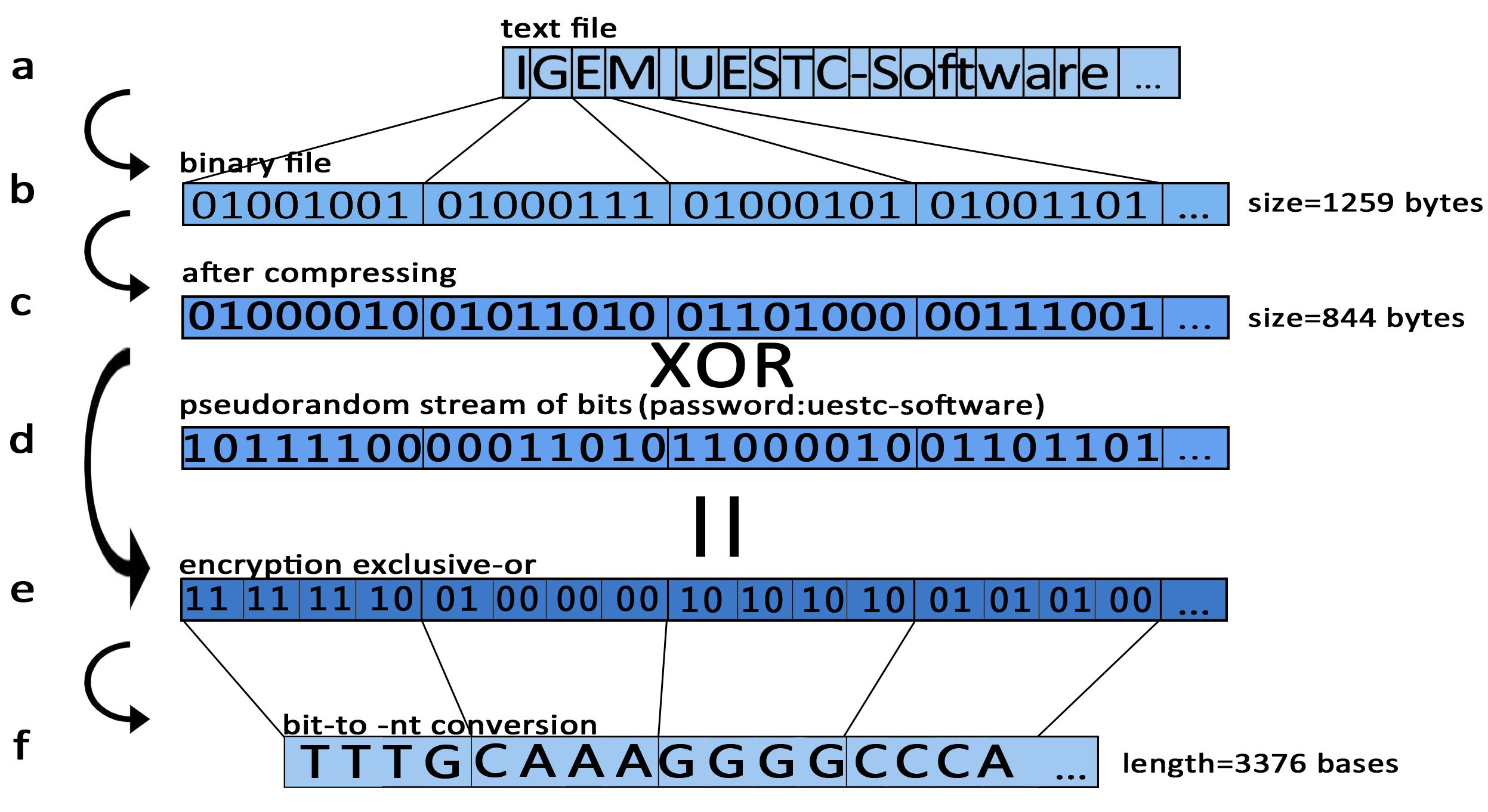Fig.4.Schematic of DNA information storage system.

The computer file (in any format, e.g. text) shown in (a) is read in binary format (b), and use bzip2 to compress it (c). Then through ISAAC encryption algorithm use pseudorandom stream of bits (d) and exclusive-or to generate a new binary string (e). Then one byte of bits converts into four bytes of A, T, C, G (e).

1. Towards the text file “IGEM UESTC-SOFTWARE”, its binary coding is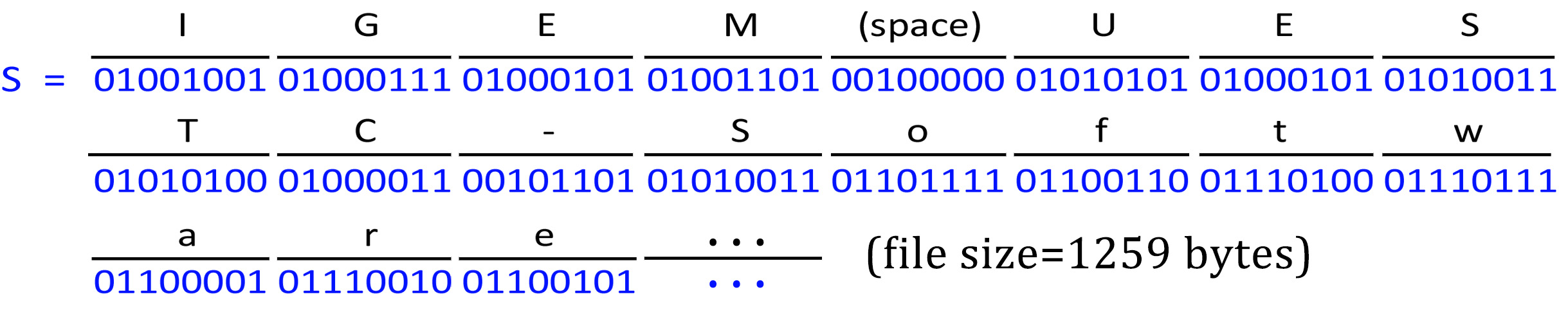2. After compressing, its compressed coding is: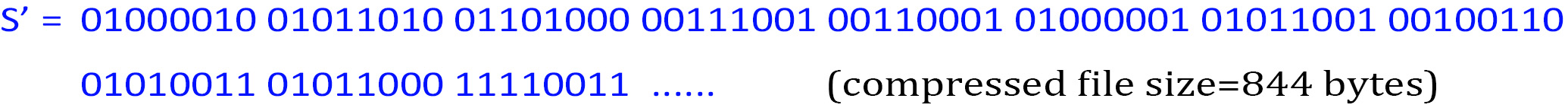3. With the pseudorandom stream of bits that ISAAC64 generated (password: uestc-software):

10111100 00011010 11000010 01101101 ......

Using XOR operation:

S’ XOR (pseudorandom stream of bits)

We get a new binary string S0: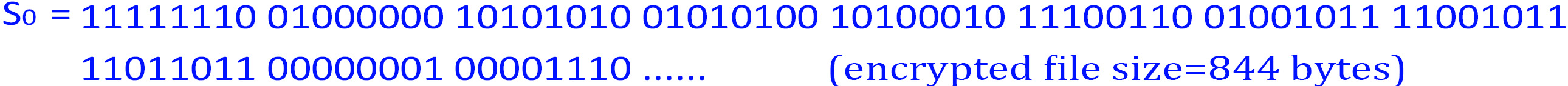4. Using the scheme illustrated in Table 1, we convert the bytes of S0 into bases—A, T, C, G.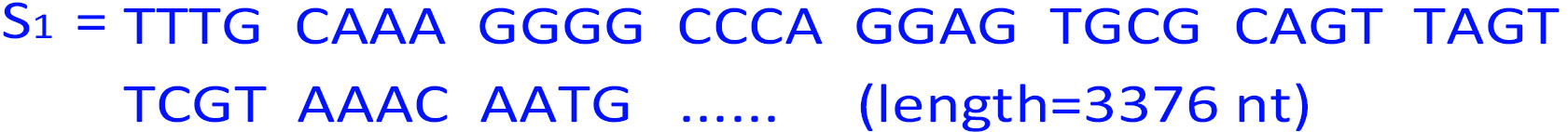5. n=len(S1 )=3376, which is 310300 in base-4. So: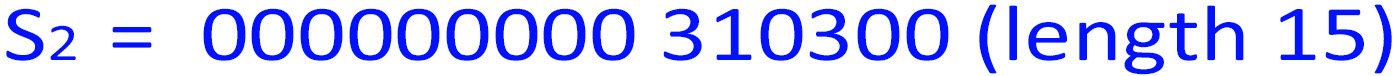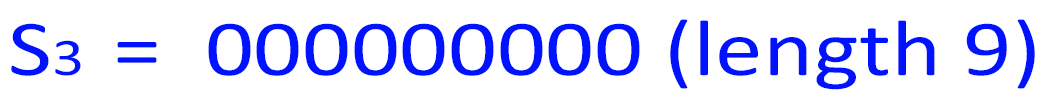len(S4 )=len(S1 )+len(S2 )+len(S3 ) = 3376 + 15 + 9 = 3400 = 50 * 68

6. Using the scheme illustrated in Table 2, convert S2 and S3 to DNA: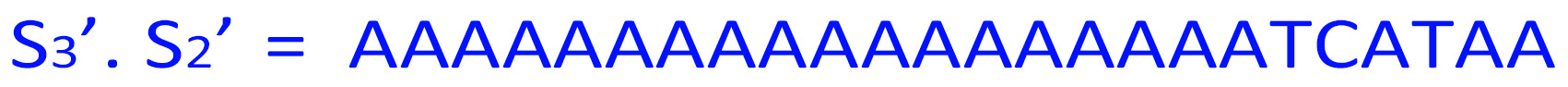Recode the DNA string S3’ . S2’ from the second character to S2’’ with repeated nucleotides as few as possible, using the scheme illustrated in Table 3.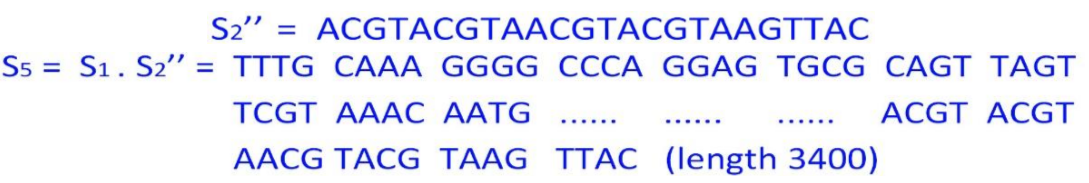7. N=len(S5 )=3400. We split S5 into overlapping segments of length 200 nt, each offset from the previous by 50 nt.

S5 will be split into overlapping segments Fi of length 200 nt for

i=0......(3400/50)-4, i=0,1,......,64

With overlapping parts underlined for illustration, F0 to Fi are: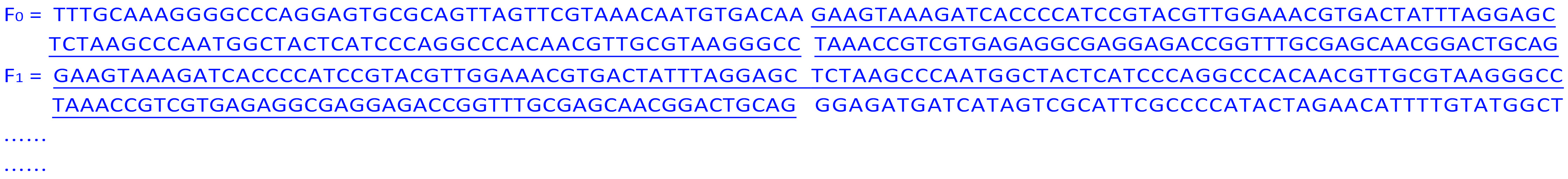8. Only i=1,3,...,63 are odd, so Fi is reverse complemented: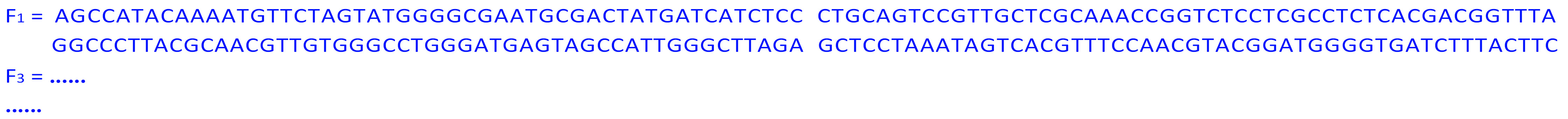9. For i=0,i4=000000000000 (length 12) and the sum (mod 4) of the even-positioned quaternaries of i4 is

P = (0+0+0+0+0+0)(mod 4) = 0

For i=1,i4=000000000001

P = (0+0+0+0+0+1)(mod 4) = 1

For i=0,IX=i4.P=0000000000000

For i=0,IX=i4.P=0000000000011.

So: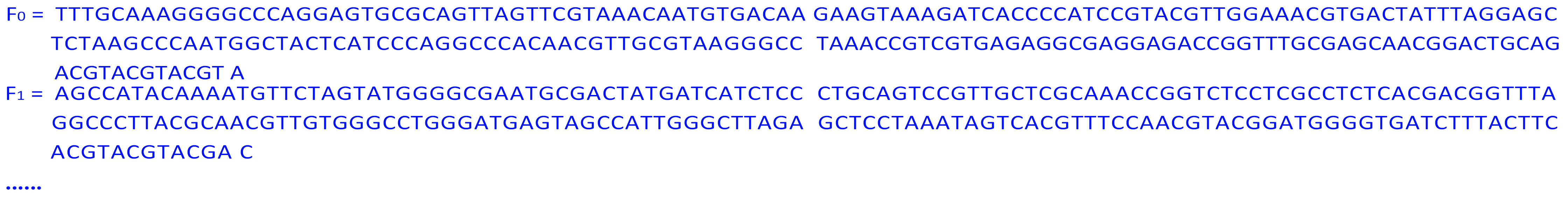11. Prepend A|T and append C|G (in this example we have three random choice, at the front of F0’’, the end of F0’’, and the end of F1’’):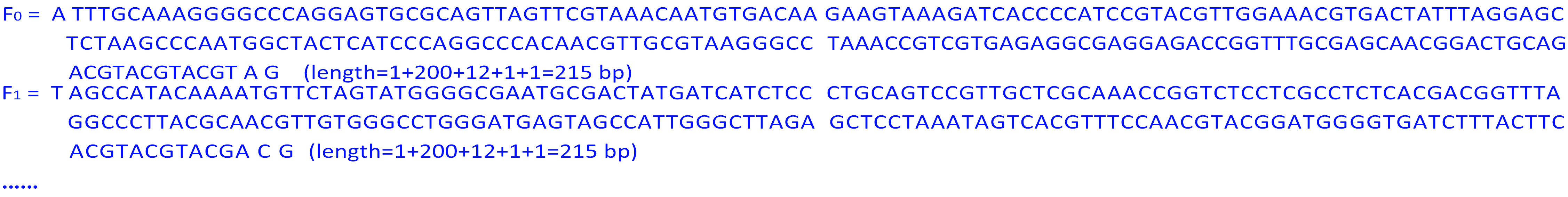## A system which enable random access and information rewriting

As a perfect information storage system, random access and information rewriting are essential. So we design another set of system which enable users recompose information stored in DNA sequences easily.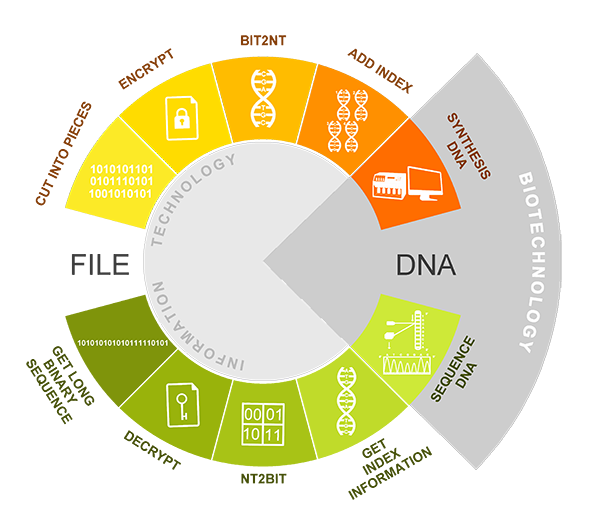Fragmenting

We read the binary code of a file, and divide the binary number into blocks of length 32 bytes.

Encrypting independently

With regard to each binary block, we use ISAAC algorithm to randomize it, which is different from encrypting the file as a whole. This ensure that we can edit the targeted data without influence other file data.

Bit-to-nt conversion

Transform each binary block to DNA sequences S1, using the same scheme in Table 1. One binary block of 32 bytes transforms into a DNA sequence of length 128 bp.

Indexing

Define

N=total number of nucleotides

With regard to each segment, conveniently indexed

i = 0,1,2,......, [N/128](7)

([ ] means round down)

Let i4 be the base-4 representation of i, appending enough leading ‘0’s so that

len(i4)=12

Recode i4 to nucleotide sequence using the same strategy above. Then compute the ‘parity quaternary’—P.

P = (i42+i44+i46+i48+i410+i412 ) mod 4

Form the indexing information string

IX=i4.P (length 13 bp)

Suffix PAM site ‘GG’ to IX, and prefix IX to each segment. (IX length = 15 bp) prepending A or T and appending C or G to each segment using the same scheme above.

One sequence length = 1+15+128+1=145 bp

Design of sgRNA

Towards the sequence needed to be edited, find the location of PAM site, and read out its downstream sequence of length 20 bp as the guide region.

To realize information edit function, we need to target and damage specific DNA segment in order to replace it by new supplement DNA. We introduce Cas9, which is an RNA-guided DNA endonuclease enzyme associated with the CRISP. SgRNA which consists of guide region (sgRNA coding aspect) and dCas9 handle(Cas9 binding aspect), can help Cas9 to identify specific DNA binding sites then guide Cas9 to bind with this site, generating a sgRNA-Cas9-dsDNA CMPX. This Cas9 cut dsDNA and destroy it. We design sgRNA’s biobrick part according to edited DNA segment, whose transcription sgRNA can guide Cas9 cut this segment specifically.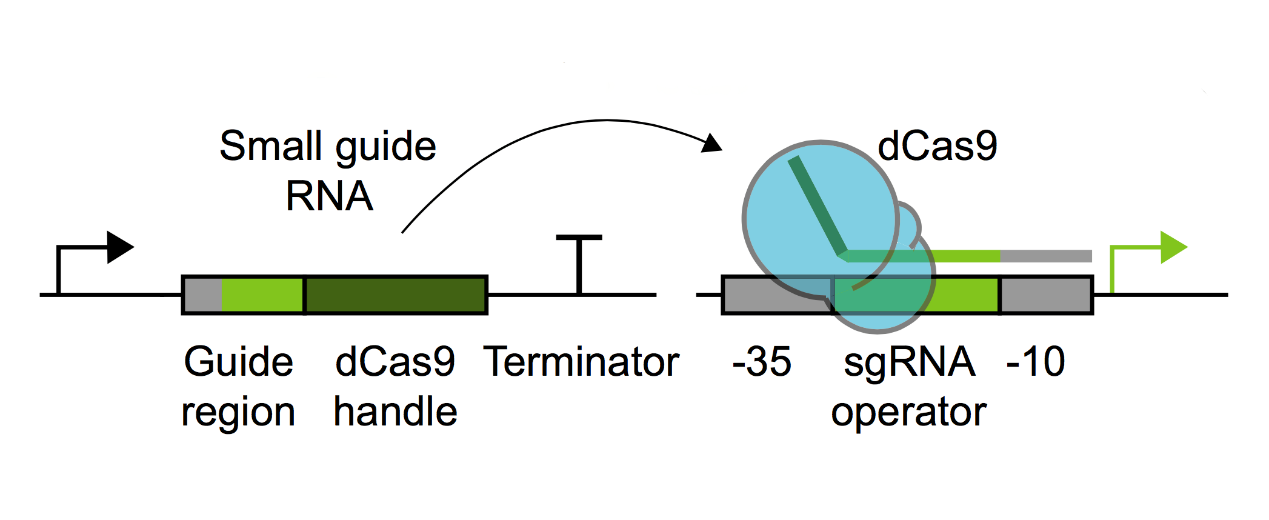•  bzip2 [online] http://www.bzip.org/
•  Robert J. Jenkins Jr. (1996) ISAAC: a fast cryptographic random number generator FSE 1996: 41-49 http://burtleburtle.net/bob/rand/isaacafa.html
•  NCBI Basic Local Alignment Search Tool [online] https://blast.ncbi.nlm.nih.gov/Blast.cgi
•  Alec AK Nielsen & Christopher A Voigt (2014) Multi-input CRISPR/Cas genetic circuits that interface host regulatory networks. Molecular Systems Biology 10: 763 | 2014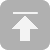CATALOGUECATALOGUE